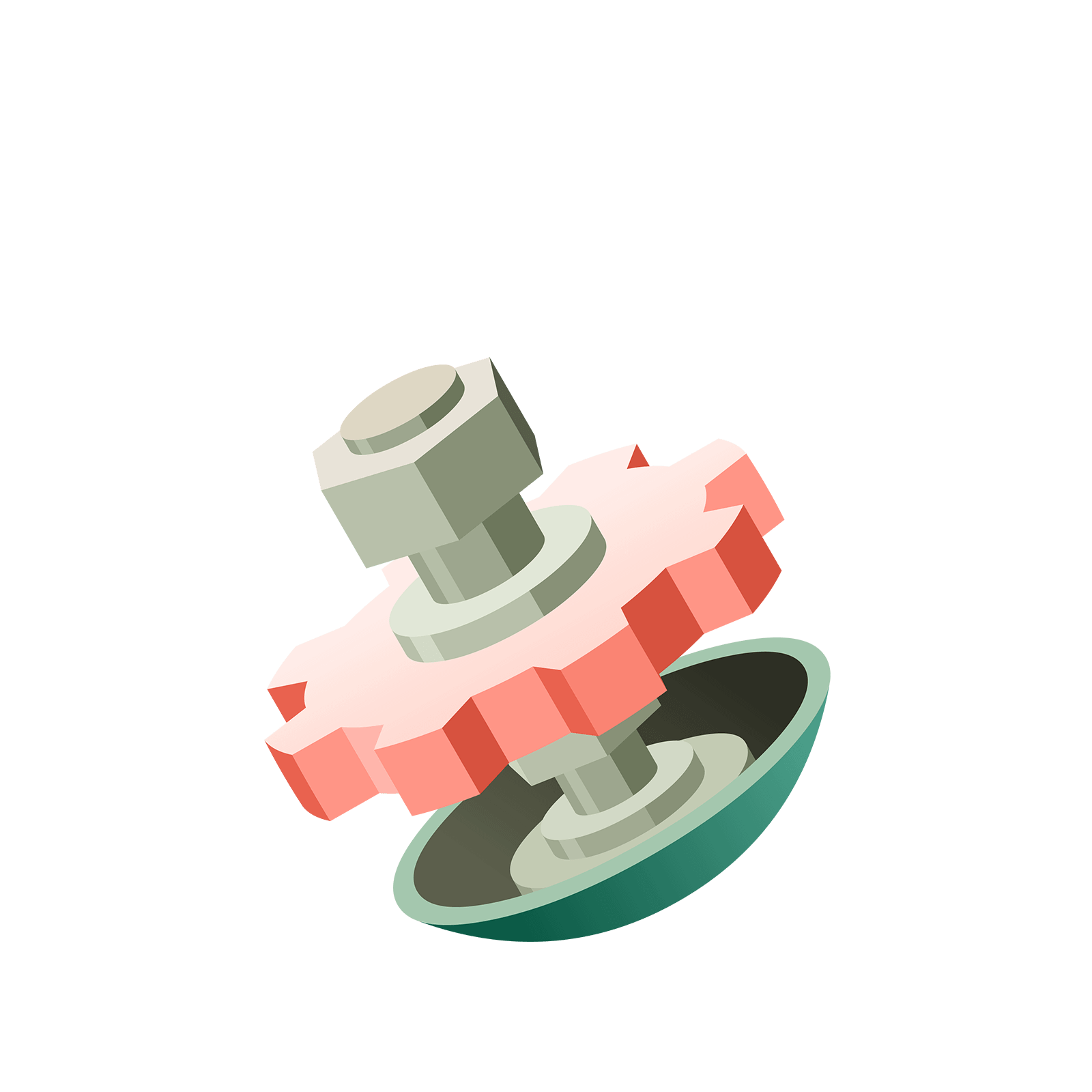05 891 995# Station  2The 1 ← 3 Machine

### Question 1

Make dots explode to find the $1 \leftarrow 3$ code for the number $13$!### Question 2

What is the code for twenty?### Question 3

In the $1 \leftarrow 3$ machine, the code $20141$ for a number is not stable.
Find a stable version of this code.

HINT: You can insert dots straight into any box you like.### Question 4

What is the value of the code $1022$ in a $1 \leftarrow 3$ machine?
Gather all the dots in the rightmost box to find out.Okay. Let's now go wild.